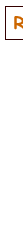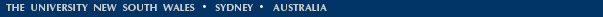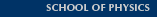*

# Open vs Closed pipes (Flutes vs Clarinets)

This page compares the acoustics of open and closed cylindrical pipes, as exemplified by flutes and clarinets, respectively. (See also Flute Acoustics and Clarinet Acoustics for introductions, or How Do Woodwind Instruments Work?) This site discusses only cylindrical pipes. Instruments such as saxophones and oboes have approximately conical bores. For the behaviour of cones compared with cylinders (and the wave patterns in flutes, clarinets, oboes etc), see Pipes and harmonics. For a background about standing waves, see Standing waves from Physclips.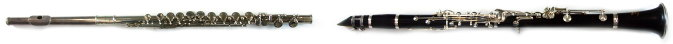The flute (photo at left) is a nearly cylindrical instrument which is open to the outside air at both ends*. The player leaves the embouchure hole open to the air, and blows across it. The clarinet (right) is a roughly cylindrical instrument which is open to the outside air at the bell, but closed by the mouthpiece, reed and the player's mouth at the other end*. The two instruments have roughly the same length. The bore of the clarinet is a little narrower than that of the flute, but this difference is not important to the argument here.

We compare open and closed pipes in three different but equivalent ways then examine some complications.

### Standing wave diagrams

First let's make some approximations: we'll pretend a flute and clarinet are the same length. For the moment we'll also neglect end corrections, to which we shall return later. The next diagram (from Pipes and harmonics) shows some possible standing waves for an open pipe (left) and a closed pipe (right) of the same length. The red line is the amplitude of the variation in pressure, which is zero at the open end, where the pressure is (nearly) atmospheric, and a maximum at a closed end. The blue line is the amplitude of the variation in the flow of air. This is a maximum at an open end, because air can flow freely in and out, and (nearly) zero at a closed end. These are what we call the boundary conditions.Open pipe (flute). Note that, in the top left diagram, the red curve has only half a cycle of a sine wave. So the longest sine wave that fits into the open pipe is twice as long as the pipe. A flute is about 0.6 m long, so it can produce a wavelength that is about twice as long, which is about 2L = 1.2 m. The longest wave is its lowest note, so let's calculate. Sound travels at about c = 340 m/s. This gives a frequency (speed divided by wavelength) of c/2L = 280 Hz. Given the crude approximations we are making, this is close to the frequency of middle C, the lowest note on a flute. (See this site to convert between pitches and frequencies, and flute acoustics for more about flute acoustics.)

The diagrams at left show that we can also fit in waves that equal the length of the flute (half the fundamental wavelength so twice the frequency of the fundamental), 2/3 the length of the flute (one third the fundamental wavelength so three times the frequency of the fundamental), 1/2 the length of the flute (one quarter the wavelength so four times the frequency of the fundamental). This set of frequencies is the (complete) harmonic series, discussed in more detail below.

(If you like, let's do the algebra: for a flute of length L, the longest wavelength (top left diagram) is λ1 = 2L and, with frequency of sound c, we have the lowest frequency f1 = c/λ1 = c/2L. The next wavelengths in the graphs at left give: f2 = c/λ2 = c/L = 2f1, then f3 = c/λ3 = c(3/2L) = 3f1, then f4 = c/λ4 = 2c/L = 4f1, and in general fn = c/λn = nc/2L = nf1, where n is a small positive integer.)

Closed pipe (clarinet). The blue curve in the top right diagram has only quarter of a cycle of a sine wave, so the longest sine wave that fits into the closed pipe is four times as long as the pipe. Therefore a clarinet can produce a wavelength that is about four times as long as a clarinet, which is about 4L = 2.4 m. This gives a frequency of c/4L = 140 Hz – one octave lower than the flute. Now the lowest note on a clarinet is either the D or the C# below middle C, so again, given the roughness of the measurements and approximations, this works out. We can also fit in a wave if the length of the pipe is three quarters of the wavelength, i.e. if wavelength is one third that of the fundamental and the frequency is three times that of the fundamental. But we cannot fit in a wave with half or a quarter the fundamental wavelength (twice or four times the frequency). So the second register of the clarinet is a musical twelfth above the first. (See clarinet acoustics for more detail.)

(So this time we have, at top right, λ1 = 4L giving lowest frequency F1 = c/λ1 = c/4L. The next wavelength is F3 = c/λ3 = c(3/4L) = 3f1, and in general Fn = c/λn = nc/4L = nf1, but only for odd n.)

* It's worth adding that the flute is not entirely open at the embouchure: the hole across which the player blows is smaller than the cross section of the pipe. This narrowing does have an acoustic effect. Nevertheless, it is sufficiently open that large oscillating flows of air can enter and leave the pipe with very little pressure difference from atmospheric. Low pressure, high flow: this boundary condition is a low value of acoustic impedance. Also, the clarinet is not completely closed by the reed: a small, varying aperture is left, even when the player pushes the reed towards the mouthpiece. However, this average area is much less than the cross section of the clarinet so the reflection of the acoustic wave is almost complete, and the acoustic flow is very small, in spite of the large acoustic pressure produced across the vibrating reed. High pressure, low flow: it is a high value of acoustic impedance. See Flute acoustics and Clarinet acoustics for details.

### Air motion animations

Some warnings about these animations. First, the density variations are not to scale: sound waves involve variations of density that are a tiny fraction of a percent. The variation shown here is vastly exaggerated to make it clear. Second, instead of using sine waves, as we did in the diagrams above, we use a very short pulse, Again, this is done for clarity. Third, we do include end corrections: the reflection at the open end occurs slightly beyond the end of the pipe (which is why the animations gradually get out of phase). Fourth, we exaggerate the amplitude of the wave that leaves the tube. In practice, only a small fraction of the energy is lost this way.

Let's send a pulse of air down a cylindrical pipe open at both ends (such as a flute, shakuhachi etc). It reaches the end of the tube and its momentum carries it out into the open air, where it spreads out in all directions. Now, because it spreads out in all directions its pressure falls very quickly to nearly atmospheric pressure (the air outside is at atmospheric pressure). However, it still has the momentum to travel away from the end of the pipe. Consequently, it creates a little suction: the air following behind it in the tube is sucked out (a little like the air that is sucked behind a speeding truck).

Now a suction at the end of the tube draws air from further up the tube, and that in turn draws air from further up the tube and so on. So the result is that a pulse of high pressure air travelling down the tube is reflected as a pulse of low pressure air travelling up the tube. We say that the pressure wave has been reflected at the open end, with a change in phase of 180°. In the open-open pipe, there is such a reflection at both ends. (This is what physicists call an 'arm-waving argument': it's neither rigorous nor quantitative. If you'd like a formal explanation, see Reflection at an open pipe.)

Now let's look at reflections in a cylindrical pipe closed at one end (an approximation of a clarinet or, better, a pipe used as a didjeridu). The reflection at an ideally closed end is easy: the high pressure pulse pushes against the closed end, which pushes back (Newton's third law): it's as though the pulse 'bounces' off the closed wall. A high pressure pulse is reflected as a high pressure pulse, with a phase change of zero in pressure.

Now compare the periods of the oscillation in these two examples and note something important: one complete cycle in the closed-open pipe (below) is four laps of the tube, and is almost twice as long as that in an open-open pipe (above), which is two laps.

A cylindrical pipe closed at both ends is rarely used deliberately in music acoustics. It has a period that is slightly shorter than does an open-open pipe. I say 'slightly' because it has no end corrections. Are there musical examples of a closed pipe? I show one of them here.

These animations were made by George Hatsidimitris.

### Frequency analysis

Now, back to comparing cylinders that are open at one end, and either open or closed at the other, like the flute and clarinet in our example.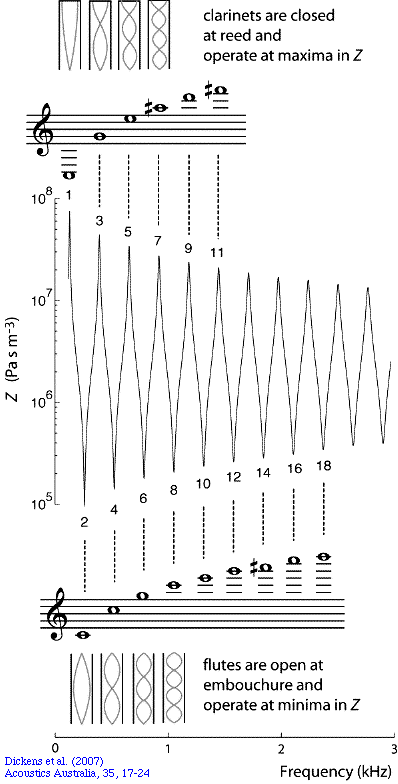The graph at right is the measured acoustic impedance of a simple cylindrical tube of length 597 mm — between the length of a flute and a clarinet — and an internal diameter of 15 mm, which is comparable with that of both. We measure at one end, and the far end is open. At low frequencies, this curve looks somewhat similar to the measured impedance of a flute with all the holes closed (Open a new window for flute lowest note response curve). This curve also looks somewhat similar (again, at low frequencies) to the measured impedance of a clarinet with all the holes closed (Open a new window for clarinet lowest note response curve). This is not so surprising because both flute and clarinet are approximately cylinders and both are open at the far end. The difference is this: the flute is open to the air at the embouchure and therefore operates at or near the minima of Z (the acoustic impedance), while the clarinet is closed and operates at or near the maxima of Z. This figure is taken from a scientific paper that explicitly compares the measured properties of clarinets and flutes with those of simple cylindrical pipes, and explains many of the complications that are discussed only briefly on this site. First let's think of this curve as the impedance spectrum for a flute. (See introduction to flute acoustics.) Looking at the minima of the spectrum, we expect that it will play at the frequencies of about 260 Hz, 520 Hz, 780 Hz, 1040 Hz, 1300 Hz etc. You can try this and find that it does: these are approximately the frequencies of the notes C4, C5, G5, C6, E6 etc - we say that it plays the harmonic series 1f, 2f, 3f, 4f etc where f is the fundamental frequency (here about 260 Hz) and the others are higher harmonics. Note that the seventh harmonic lies between A and A#, as indicated. If you don't have a flute or clarinet handy, you can listen to the sound files below. Let's now think of the graph above as the impedance spectrum of a clarinet with all tone holes closed. (See introduction to clarinet acoustics.) The pipe used is slightly longer than the cylindrical part of the bore of the clarinet, so this calculation will be approximate. Looking at the maxima of the spectrum, we expect that it will play at frequencies of about 130 Hz, 390 Hz, 650 Hz. So this hypothetical (cylindrical) clarinet with all its holes closed plays a series of notes C3, G4, E5 and some squeaks above that. In other words the series 1F, 3F, 5F etc where F is the fundamental frequency, which is about 130 Hz for the hypothetical clarinet with all holes closed. (In practice, the A clarinet plays a series starting on C#3 and the Bb clarinet D3 and higher notes, because their cylindrical sections are shorter than the flute. This issue is further complicated because A and Bb clarinets are transposing instruments, and so clarinettists give these notes names a minor third or a major second higher, respectively. There are complications due to end corrections, and also to the fact that the clarinet is rather less cylindrical than a flute: it has a flare and a bell at one end, and a strong taper in the mouthpiece at the other. (For a discussion of these differences, see the scientific paper.)

### The overtone series on the flute and clarinet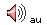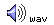Overblowing the lowest note on a flute.
For the flute, the notes played by overblowing fall close to the harmonics of the lowest note. This is not the case for the clarinet.Overblowing the lowest note on a clarinet.

The notes in the diagram are the harmonics. The notes in the sound file are the notes played by overblowing, without using register keys. With the exception of 3f0, the played notes are considerably flatter than the harmonic frequencies. This effect is due to the fact that the instrument is not a simple cylinder. This is discussed in Introduction to clarinet acoustics and in the paper whence these figures come.

The harmonic series of notes is explained in terms of the standing waves of the instrument on the page Pipes and harmonics. This page also explains why sound spectra of notes in the low range of the clarinet have only weak even harmonics in their sound spectra. Further it explains the case of the conical instruments (oboe, bassoon, saxophone): an oboe is roughly as long as a flute and a clarinet, and it is closed like the clarinet, but it plays roughly the same range as the flute and has all harmonics present.

### End effects and end corrections

First, look closely at the animation in the section above about reflections at an open end of a pipe. The reflection is caused when a pulse of high pressure air gets to the end of the pipe and it spreads out. But what happens exactly at the end? Inside the tube there is a plane wave, and when the wave is radiating externally it is a spherical wave, but between the two there is some complicated geometry. In this phase, the pulse of air is neither in the free, unimpeded air away from the pipe, nor in the tightly constrained environment of the pipe. It is somewhere between the two: unconstrained on one side, but constrained by the pipe on the other.

As we explain above, the reflection is caused by suction that results when the momentum of the pulse of air takes it away from the pipe. This suction doesn't appear immediately when the pulse reaches the end of the pipe, but a little later, as it starts to spread out.

So the reflection appears to occur slightly beyond the open end of the pipe. To a rather good approximation, this effect can be calculated by assuming that the effective length of the pipe is a little longer than its geometrical length. The difference is called the end correction. It makes the pipe resonance occur at a slightly lower frequency than a naive model, with no end effect. Here's another way to think of this: the wave in the pipe must accelerate a small volume of mass just outside the end of the pipe. This air has mass, and adding that extra mass lowers the resonant frequency of vibration.

For a simple cylindrical pipe as shown above, experiments and calculations show that the end effect (or end correction) at the open end is equivalent to increasing the pipe by a length of about 0.6 times the radius. Note the consequence of this: all else equal, a large diameter pipe is a little flatter than a thin one. For a closed end, there is no such end correction.

If you look closely at the animations above, you'll see that we have included end effects. Although the geometrical lengths of the two pipes are equal, the open-open pipe has two end corrections and so its effective length is slightly greater than that of the open-closed pipe. Hence the travelling pulses get successively further out of step with round-trip through the pipes.

### Real instruments: further complications!Real flutes and clarinets are of course more complicated than simple cylinders. For the purposes of this discussion, the greatest complication is caused by the presence of tone holes and keys. To a crude approximation, opening all or most of the holes below a certain point on the instrument shortens the pipe available for standing waves. At low frequencies, the instrument behaves somewhat like a cylindrical pipe with an 'effective length' determined by the position of the first open key. That real life is more complicated is demonstrated by the two sound spectra at right. One is a flute playing the note D5, the other is a clarinet playing the note D5 (called E5 on a Bb clarinet). In each case, the microphone was at 3 cm from the first open tone hole. Can you tell which is which? This figure is taken from a scientific paper, which deals with some of these complications, and explains some of the differences and similarities between flutes and clarinets, and compares them in detail with simple pipes. (And gives the answer to the question.)

### Higher resonances in the time domain

Taking the (corrected) pipe length as L and the speed of sound as c, the open-open pipe has its first resonance at c/2L and its second resonance at c/L. The closed-open pipe has its first two resonances at c/4L and 3c/4L. So it's nice to see animations of these in the time domain, to compare with those above. Here they are for the second resonances of open and closed pipes.

 Your browser does not support the video tag.

For a simplified introduction to the whole family, see How Do Woodwind Instruments Work? (That page also has a simple introduction to sound waves.)[Basics | Research | Publications | Flutes | Clarinet | Saxophone | Brass | Didjeridu | Guitar | Violin | Voice | Cochlear ]
[ People | Contact Us | Home ]

Joe Wolfe / J.Wolfe@unsw.edu.au
phone 61-2-9385 4954 (UT + 10, +11 Oct-Mar)
Joe's music site### Happy birthday, theory of relativity!

As of June 2005, relativity is 100 years old. Our contribution is Einstein Light: relativity in brief... or in detail. It explains the key ideas in a short multimedia presentation, which is supported by links to broader and deeper explanations.